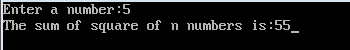Sum of squares of a given list of numbers

THEORY:
The sum of the series (1^2 + 2^2 + 3^2 + ... + N^2) is
(N * (N + 1) * (2N + 1)) / 6

CODING:
#include<iostream.h>
#include<conio.h>
void main()
{
int a=1,sum=0,n;
clrscr();
cout<<"Enter a number:";
cin>>n;
while(a<=n)
{
sum=sum+a*a;
a++;
}
cout<<"The sum of square of n numbers is:"<<sum;
getch();
}
OUTPUT: